# Boundary Layer Flow of Silver and Titaniumoxide Nanofluids over Vertical Stretching Sheet

Boundary Layer Flow of Silver and Titaniumoxide Nanofluids over Vertical Stretching Sheet

PragyaR. Vasanthakumari

Research scholar, Dept. of Mathematics, Bharathiar university, Coimbatore

Associate Professor, Dept of Mathematics, KMCPG studies, Pondicherry

Corresponding Author Email:
pragyapondy@gmail.com
Page:
371-376
|
DOI:
https://doi.org/10.18280/ijht.340304
|
Accepted:
|
Published:
30 September 2016
| Citation

OPEN ACCESS

Abstract:

The present paper focuses on the convective heat transfer of boundary layer flow of incompressible, viscous Nano fluids over a vertical linear stretching sheet. Two types of nanofluids such as silver - Water nanofluid and titanium oxide -Water nanofluid are considered for the present study. Various physical parameters for both types of Nanofluids for different volume fractions are calculated. The Boundary layer equations of motion and energy which are non-linear partial differential equations are reduced to non-linear ordinary differential equations by means of similarity transformations. The resulting non-linear ordinary differential equations are solved numerically by most efficient Nachtsheim-Swigert shooting iteration technique for satisfaction of asymptotic boundary conditions along with Fourth Order Runge-Kutta Integration Method. This study analyzes the effectiveness of heat transfer of Nano fluids in cooling plastic and rubber sheet.

Keywords:

Nano fluids, Nanoparticles, Boundary layer equation, Stretching sheet.

1. Introduction

The cooling and heating effects are required by power manufacturing, transportation, electronics etc. These cooling and heating techniques are greatly needed for high energy device. It was clear that common ﬂuids have limited heat transfer capabilities due to their low heat transfer capacity. However some metals are found to have very high thermal conductivity, may be three to four times higher than the common ﬂuids. Therefore it is required to make a substance by combining these two, which will behave like a ﬂuid and have thermal conductivity of a metal. Nanoﬂuids are such substances, made by suspending the nano particles (with diameter 1 to 100 nanometer) in the common ﬂuids, called base ﬂuids. A very small amount of these nanoparticles in the base ﬂuids increases the thermal conductivity by 15-40%.

Nanofluid, a name conceived by Choi at Argonne National laboratory, are ﬂuids consisting of solid nanoparticles with size less than 100 nm suspended with solid volume fraction typically less than 4%. A nanoﬂuid can enhance heat transfer performance compared to pure liquids. Nanoﬂuids can be used to improve thermal management system in many engineering application such as transportation, micromechanics and instrument, HVAC system and cooling devices. Recently, many investigators studied nanoﬂuid convective heat transfer in different geometry both numerically and experimentally.

Nanofluids are helpful in many areas of medical application like cancer therapy and laser based surgery which involves cooling of equipment.Nanofluid technology can be effectively used for cooling of powerful and small computers and other electronic devices. It can be also used for large scale cooling like aeroplanes, military system. Nanofluids can be widely used in large scale industries like chemical, food and beverages, oil, paper, textiles etc.

Das et al.  experimentally showed a two - to four - fold increase in thermal conductivity enhancement for water-based nanofluids containing Al2O3 or CuO nanoparticles over a small temperature range, 21o – 51oC. A comprehensive survey of convective transport in nanofluids has been made by Buongiorno , who gave a satisfactory explanation for the abnormal increase of the thermal conductivity. Buongiorno and Hu , studied on the nanofluid coolants in advanced nuclear systems. Ahmad and Pop  investigated mixed convection boundary layer flow from a vertical flat plate embedded in a porous medium filled with nanofluids. Boundary layer flow of nanofluids over a moving surface in a flowing fluid was examined by Bachok et al. . Khan and Pop  discussed the boundary-layer flow of a nanofluid past a stretching sheet. Makindeand Aziz  explained the boundary layer flow of a nanofluid past a stretching sheet with a convective boundary condition. Daungthongsuk and Wongwises  studied analytically the effect of thermo physical properties models, for predicting the convective heat transfer rate for low concentration nanofluid.

Laminar boundary layer flow of nanofluid along a flat plate was studied by SP Anjali Devi and Juljia Andrews . Vajravelu et al.  studied the effect of convective heat transfer in the flow of viscous Ag-water and Cu-water nanofluids over a stretching surface and it was seen that the role of nanoparticle volume fraction on the flow and heat transfer characteristics under the influence of thermal buoyancy and temperature dependent internal heat generation and absorption. Natural convection over vertical plate of nanofluid have been explained by Reddy et.al. . Abdel Malik Bouchouchaand, Rachid Bassiah studied nanofluids in square cavity .

In this study, our objective is to investigate the effect of viscous nanofluid past over vertical stretching surface. Here the two nanofluids considered are Silver-water nanofluid and Titanium oxide-water nanofluid. Using an appropriate similarity transformation, the well-known governing partial differential equations are reduced to the ordinary differential equations. Numerical solution of the problem is obtained using Nachtsheim-swigert shooting iteration scheme for satisfaction of asymptotic boundary conditions along with Fourth order Runge-Kutta integration method. These numerical results for various physical parameters involved in the problem are demonstrated graphically. The skin friction coefficient and the non-dimensional rate of heat transfer are also presented numerically in tabular form for several values of the physical parameters.

2. Formulation of Problem

Consider a two - dimensional, steady, incompressible nanofluid past over vertical stretching sheet. The velocity of the stretching sheet is given by u = ax where a> 0, is constant acceleration parameter. X –axis is taken along the direction of stretching sheet. The flow is generated by the stretching sheet along X axis.

Using Prandtl boundary layer equations based on principle of conservation of mass, energy and momentum, equations of nanofluids is given by

$\frac{\partial u}{\partial x}+\frac{\partial v}{\partial y}=0$ (1)

$u \frac{\partial u}{\partial x}+v \frac{\partial u}{\partial y}=\vartheta_{n f} \frac{\partial^{2} u}{\partial y^{2}}$ (2)

$u \frac{\partial T}{\partial x}+v \frac{\partial T}{\partial y}=\alpha_{n f} \frac{\partial^{2} T}{\partial y^{2}}$ (3)

Components of velocity along x and y directions are represented by u and v respectively, T is temperature, $\boldsymbol{\vartheta}_{\boldsymbol{n} \boldsymbol{f}}$ is kinematic viscosity of nanofluids, $\alpha_{n f}$ is thermal diffusibility of nanofluids, knf thermal conductivity, (Cp)nf is specific heat and ρnf density of nanofluids.

$y=0, u=u_{w}, v=0, T=T_{w}+\mathbf{a} \mathrm{x}$

$y \rightarrow \infty, u \rightarrow 0, T \rightarrow T_{\infty}$ (4)

The viscosity, heat capacity and thermal conductivity of the nanofluids depends upon volume fraction $\phi$ of nanoparticles used. The effective density of nanofluid is given by

$\rho_{n f}=(1-\emptyset) \rho_{f}+\rho_{s}$ (5)

And heat capacitance of nanofluid

$\left(\rho C_{p}\right)_{n f}=(1-\emptyset)\left(\rho C_{p}\right)_{f}+\emptyset\left(\rho C_{p}\right)_{s}$ (6)

Thermal expansion coefficient of nanofluid is given by

$(\rho \beta)_{n f}=(1-\phi)(\rho \beta)_{f}+\phi(\rho \beta)_{s}$ (7)

As given by Santraet al. (2009), where ρf and ρs are density, (Cp)f and (Cp)s are specific heat capacitance of base fluid and solid particle respectively and βf and βs are thermal expansion coefficient of base fluid and solid particle respectively.

The dynamic viscosity of nanofluids as given by (Brinkman 1952) is as follows:

$\mu_{n f}=\frac{\mu_{f}}{(1-\emptyset)^{2.5}}$(8)

$\frac{k_{n f}}{K_{f}}=\frac{\left(k_{S}+2 k_{f}\right)-2 \emptyset\left(k_{f}-k_{S}\right)}{\left(k_{S}+2 k_{f}\right)+\emptyset\left(k_{f}-k_{S}\right)}$ (9)

Table 1. Density, capacitance and thermal expansion coefficient water, silver, titanium oxide

 (kg/m3) Cp (J/ kg.K) K (W/m.K) Water 997.1 4179 0.613 Silver 10,500 234 429 Titanium oxide 4,2500 686.2 8.9532

To solve the equations following dimensionless variables are introduced:

$\psi=\left(a \vartheta_{n f}\right)^{1 / 2} x f(\eta)$ (10)

$\eta=\left(\frac{a}{\vartheta_{n f}}\right)^{1 / 2} y$ (11)

$u=a x f^{\prime}(\eta)$ (12)

$v=-\left(a \vartheta_{n f}\right)^{\frac{1}{2}} f(\eta)$ (13)

where $\Psi$(x, y) is stream function and $u=\frac{\partial \psi}{\partial y}$ and $v=-\frac{\partial \psi}{\partial x}$

, η is similarity variable. And,

$\theta(\eta)=\frac{T-T_{\infty}}{T_{w}-T_{\infty}}$ (14)

Continuity equation is satisfied and equations (2) and (3) along with boundary conditions. (4) are transformed and are written as:

$f^{\prime \prime \prime}-(1-\emptyset)^{2.5}\left(1-\emptyset+\emptyset \frac{\rho_{s}}{\rho_{f}}\right)\left(f^{\prime 2}-f f^{\prime \prime}\right)+$$\lambda \theta(1-\emptyset)^{2.5}\left(1-\emptyset+\emptyset \frac{(\rho \beta)_{s}}{(\rho \beta)_{f}}\right)=0$ (15)

$\frac{1}{\operatorname{Pr}_{n f}} \frac{k_{n f}}{k_{f}} \theta^{\prime \prime}+\left(1-\emptyset+\emptyset \frac{\rho_{s}}{\rho_{f}}\right)\left(1-\emptyset+\emptyset \frac{\left(\rho c_{p}\right)_{s}}{\left(\rho c_{p}\right)_{f}}\right) f \theta^{\prime}=0$ (16)

Along with boundary conditions:

$\eta=0, y=0, f=0, f^{*}=1, \theta=1$

$\eta \rightarrow \infty, y \rightarrow \infty, f^{\prime}=0, \theta=0$ (17)

The variables are defined as

$\operatorname{Pr}_{n f}=\frac{\vartheta_{n f}}{\alpha_{n f}}$ (18)

Kinematic viscosity and thermal diffusivity of nanofluids are respectively:

$\vartheta_{n f}=\frac{\mu_{n f}}{\rho_{n f}}$ (19)

and

$\alpha_{n f}=\frac{k_{n f}}{\rho_{n f(C p)_{n f}}}$ (20)

And thus (Pr)nf, the Prandtl number of nanofluid

$Pr_{nf}=\mu\frac{(Cp)_{nf}}{k_{nf}}$   (21)

where $λ=\frac{g(ρβ)f}{ρfa^2}$, $Pr=\frac{\upsilon f}{\alpha f}$.

Skin friction coefficient:

The skin friction coefficient cf is given by $C_f=\frac{\tau w}{_{ρf}U^2_w}$, where $\tau_w=\mu_{nf}(\frac{\partial u}{\partial y})_{y=0}$. Using (11), we get $C_fRe^{1/2}_x=\frac{1}{(1-∅)^{2.5}}f''(0)$.

Nusselt number:

Nusselt number is defined by: $Nu=\frac{xqw}{k_f(T_w-T_f)}$ Where $q_w=-k_{nf}(\frac{\partial T}{\partial y})_{y=0}$ Using (11) we get $NuRe^{-1/2}_x=-\frac{k_{nf}}{K_f}\theta'(0)$.

3. Solution of Problem

To solve equations (16) and (17) along with boundary conditions (18), shooting technique along with Runge – Kutta method of fourth order is used. Initial guesses were made for values of f’’ to start shooting process as given by MA Satar and Maleque in 2011. The value of η is found on each iteration loop by ηn+1 n+∆η. The step size ∆η = 0.001 is used while obtaining the numerical solution with η max = 15 and by considering the six decimal place as a criterion for convergence.

4. Results and Discussions

In this section, we consider two types of water based nanofluids containing Silver(Ag) and Titanium oxide (Ti2O3). The Prandtl Number of the base fluid (Water) is kept constant at 7.2 and the effect of solid volume fraction φ is investigated in the range of 0≤∅≤0.2 . In order to get the clear insight of the problem, numerical values of the solutions are obtained by fixing various values for the physical parameters involved having φ = 0.0 for base fluid and  φ = 0.1 for the nanofluid when λ = 1.0. The results are exhibited through the graphs and tables. The heat transfer property of nanofluid over a vertical stretching sheet has been studied. Table 2 and 3 depicts density, capacitance, dynamic viscosity, thermal conductivity, Prandtl number of silver water and titanium oxide water nanofluids.

The study basically concentrates on flow of silver water and titanium oxide water nanofluids over stretching sheet. Results are shown for various physical parameters. Effect of heat transfer by changing volume fractions and Prandtl number have been analysed. The effect of change of volume fraction on various physical parameters has been depicted by graphs. Increase in volume fraction leds to increase in temperature profile of both types of nanofluids.

Figure 1 and 2 represents dimensionless temperature profile of silver water and titanium oxide water nanofluids respectively. It is noticed that increase in Prandtl number leds to decrease in temperature.

Figure 3 shows the effect of volume fraction on temperature profile of Titanium oxide nanofluids. It is observed that increase in volume fraction causes increase in temperature profile. There is very less effect of volume fraction on temperature.

Figure 4 depicts temperature profile of silver water and titanium oxide water nanofluids with Prandtl number 2.37 and 6.66 respectively. Increse in Prandtl number leads to decrease in temperature.

Figure 5 shows change in values of –θ’(0) with different Prandtl numbers.-θ’(0) denotes local heat transfer. It is noticed that Prandtl number increases as local heat transfer decreases.

Figure 6 shows change in volume fraction leds to volume fraction leds to change in Prandtl number. It is observed that with increase in volume fraction, Prandtl number decreases for both types of nnofluids. Decrease in Prandtl number of silver water nanofluid is more in comparison to titanium oxide nanofluid.

Figure 7 shows change in volume fraction causes change in density for both types of nanofluids. Increase in volume fraction increases density. Increse in density is more for silver water nanofluid than Titanium oxide nanofluids.

Hence following conclusions may be drawn:

• Nanofluids are better coolants than their base fluid as they have higher thermal conductivity.
• The increase in Prandtl number cause decrease in temperature for both silver water and titanium oxide water nanofluids.
• Both Prandtl number and heat capacity decreases with increase in volume fraction for both types of nanofluids
• Changing volume fraction also changes density and thermal conductivity for both types of nanofluids. Increase of volume fraction increases density and thermal conductivity and vice versa.
• Further, increase in volume fraction causes increase in heat transfer coefficient for both types of nanofluids.
• Viscosity remains unaltered for different types of nanofluids. However, increasing volume increase viscosity for both types of nanofluids.

Table 2. Thermophysical properties of silver Nanofluids

 $\varPhi$ $\rho$nf (Cp)nf $\mu$nf Knf (Pr)nf 0.00 997.1 4179 0.001002 0.5970 7.02 0.01 1092.129 4139.55 0.001027 0.6556 6.05 0.02 1187.158 4100.1 0.001053 0.7161 5.01 0.03 1282.187 4060.65 0.001081 0.7858 4.21 0.04 1377.216 37550.82 0.01109 0.8488 4.02 0.05 1472.245 3981.75 0.001139 0.9432 3.66 0.06 1567.274 3942.3 0.001169 0.9991 3.17

Table 3. Thermophysical properties of Titanium oxide Nanofluids

 $\varPhi$ $\rho$nf (Cp)nf $\mu$nf Knf (Pr)nf 0.00 997.1 4179 0.001002 0.5970 7.02 0.01 1029.629 4144.072 0.001027 0.6153 6.92 0.02 1062.158 4109.144 0.001053 0.6428 6.84 0.03 1094.687 4074.216 0.001081 0.6596 6.76 0.04 1127.216 4039.288 0.01109 0.6701 6.69 0.05 1159.745 4004.36 0.001139 0.6902 6.56 0.06 1192.274 3969.432 0.001169 0.7013 6.43

## figure_1.jpg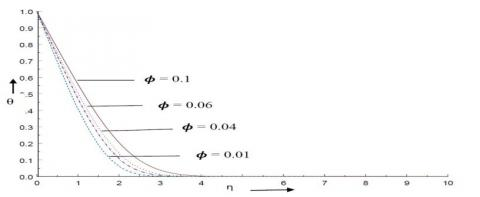Figure 1. A comparative study of volume fraction against temperature profile for silver water nanofluid

## figure_2.jpg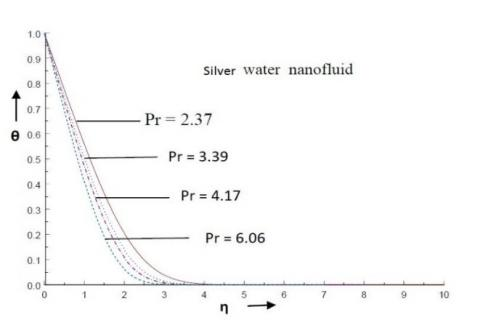Figure 2. Temperature profile for various values of Pr no. of silver water nanofluid.

## figure_3.jpgFigure 3. A comparative study of volume fraction against temperature profiles of Titanium oxide water nano fluid

## figure_4.jpg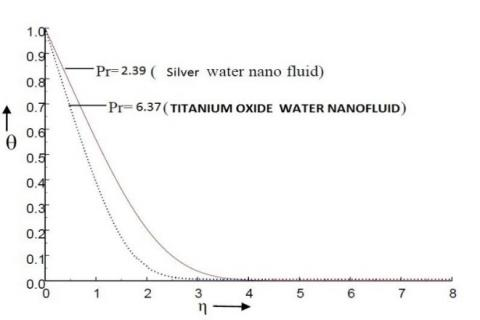Figure 4. Temperature profiles of silver water and titanium water nanofluid

## figure_5.jpg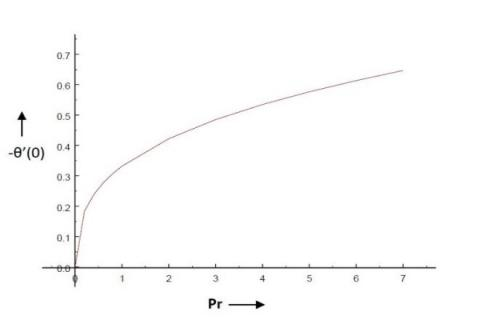Figure 5. Effect of Prandtl number on local heat transfer

## figure_6.jpgFigure 6. Prandtl number for different values of volume fraction for silver-water and titanium oxide water nanofluid

## figure_7.jpg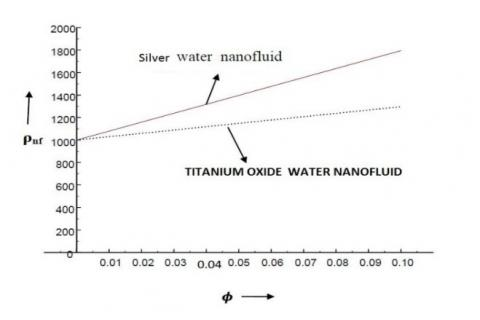Figure 7. A comparative study of effect of volume fraction against density for silver-water and titanium oxide water nanofluid

Nomenclature

Cp        Specific heat

$\rho$           Density of fluid

Β          Thermal expansion coefficient

T           temperature

G          Acceleration due to gravity

K          thermal conductivity

Nu        local Nusselt number

Re        Reynold’s number

Α          thermal diffusiblity

Β          thermal expansion coefficient

$\varPhi$          solid volume fraction

$\Theta$          dimensionless temperature

η           similarity variable

$\mu$          dynamic viscosity

f           fluids

nf         nanofluids

s           solid particles

References

 Brinkman, H. C. “The viscosity of concentrated suspension and solutions,” J.Chem Phys., vol. 20 pp. 571-581, 1952.

 Das S. K., Putra N., Thiesen P., and Roetzel W., “Temperature dependence of thermal conductivity enhancement for Nanofluids,” J. Heat Transfer, vol. 125, pp. 567–574, 2003. DOI: 10.1115/1.1571080.

 Buongiorno J., “Convective transfer in Nanofluids,” ASME J. Heat Transfer, vol. 128, pp. 240–250, 2006. DOI: 10.1115/1.12150834.

 Buongiorno J. and Hu, W., “Nanofluid coolants for advanced nuclear power plants.” Paper no. 5705, Proceedings of ICAPP ‘05, Seoul, May 15–19, 2005.

 Ang, X. Q. & Mujumdar, A. S., “Heat transfer characteristics of nanofluids: A review.” Int. J. Thermal Sciences, vol.46, pp.1-19, 2007. DOI: 10.1016/j.ijthermalsci.2006.06.010.

 Ahmad S. and Pop I., “Mixed convection boundary layer flow from a vertical plate embedded in a porous medium filled with nanofluids.” International Communications in Heat and Mass Transfer, vol. 37, pp. 987-991, 2010. DOI: 10.4236/anp.2016.51014.

 Bachok N., Ishak A. and Pop I., “Boundary layer flow of nanofluids over a moving surface in flowing fluid,” International Journal of Thermal Sciences, vol. 49, pp. 1663-1668, 2010.

 Khan W. A. and Pop I., “Boundary layer flow of a nanofluid past a stretching sheet,” International Journal of Heat and Mass Transfer, vol. 53, no. 11-12, pp. 2477–2483, 2010.

 Makinde O. D. and Aziz A., “Boundar layer flow of nanofluid past a stretching sheet with convective boundary condition,” International Journal of Thermal Sciences, vol. 50, no. 7, pp. 1326–1332, July 2011.

 Daungthongsuk W. and Wongwises S., “Effect of thermophysical properties models on the predicting of the convective heat transfer coefficient,” International Communications in Heat and Mass Transfer, vol. 35, pp.1320-1326, 2008.

 S. P. Anjali Devi and Julie Andrews, “Laminar boundary layer flow of nanofluid over a flat plate,” Int. J. of Appl. Math and Mech., vol. 7, no. 6, pp. 52-71, 2011.

 Vajravelu K., Prasad K. V., Lee J., Lee C. Pop I. and Van Gorder R. A., “Convective heat transfer in the flow of viscous Ag-water and Cu-water nanofluids over a stretching surface,” Int J Therm Sci, vol. 50, pp. 843–851, 2011.

 N. F. M. Noor, S. Abbasbandy and I. Hashim, “Heat and mass transfer of thermophoretic MHD flow over an inclined radiate isothermal permeable surface in the presence of heat source/sink,” International Journal of Heat and Mass Transfer, vol. 55, pp. 2122-2128, 2012.

 T. G. Motsumi and O. D. Makinde, “Effects of thermal radiation and viscous dissipation on boundary layer flow of nanofluids over a permeable moving flat plate,” Phys Scr, vol. 86, 2012.

 O. D. Makinde, W. A. Khan and Z. H. Khan, “Buoyancy effects on MHD stagnation point flow and heat transfer of a nanofluid past a  convectively heated stretching/shrinking sheet,” Int J Heat Mass Transfer, vol. 62, pp. 526–533, 2013.

 O. D. Makinde, “Computational modelling of nanofluids flow over a convectively heated unsteady stretching sheet,” Curr Nanosci, vol. 9, pp. 673–678, 2013.

 S. Das, R. N. Jana and O. D. Makinde, “MHD Boundary layer slip flow and heat transfer of nanofluid past a vertical stretching sheet with non-uniform heat generation/absorption,” Int J Nanosci, vol. 13, 2014.

 Mohsen Sheikholeslami, Davood Domiri Ganji, M. Younus Javed and R. Ellahi, “Effect of thermal radiation on magneto hydrodynamics nanofluid flow and heat transfer by means of two phase model,” J. Magn Magn Mater, vol. 374, pp. 36–43, 2015.

 S. Nadeem, Rashid Mehmood and Noreen Sher Akbar, “Partial slip effect on non-aligned stagnation point nanofluid over a stretching convective surface,” Chin Phys B, vol. 24, no. 1, 2015.

 S. Nadeem, R. Mehmood and N. S. Akbar, “Oblique stagnation point flow of CNT based fluid over a convective surface,” Comput Theor Nanosci, vol. 12, pp. 1–8, 2015.

 S. Nadeem, R. Mehmood and S. S. Motsab, “Numerical investigation on MHD oblique flow of a Walter’s B type nano fluid over a convective surface,” Int J Therm Sci, vol. 92, no. C, pp. 162–172, 2015.

 S. Nadeem, R. Mehmood and N. S. Akbar, “Combined effects of magnetic field and partial slip on obliquely striking rheological fluid over a stretching surface,” J Magn Magn Mater, vol. 378, pp. 457–462, 2015.

 S. Nadeem, R. Mehmood and Noreen Sher Akbar, “Thermo-diffusion effects on MHD oblique stagnation-point flow of a viscoelastic fluid over a convective surface,” Eur Phys J Plus, vol. 129, no. 182, pp. 1–18, 2014.

 R. Ellahi, S. Aziz and A. Zeeshan, “Non Newtonian nanofluids flow through a porous medium between two coaxial cylinders with heat transfer and variable viscosity,” J Porous Media, vol. 16, no. 3, pp. 205–216, 2013.

 Mohsen Sheikholeslami, Davood Domiri Ganji, M. Younus Javed and R. Ellahi, “Effect of thermal radiation on magneto hydrodynamics nanofluid flow and heat transfer by means of two phase model,”  J. Magn Magn Mater, vol. 374, pp. 36–43, 2015.

 M. Sheikholeslami, M. Gorji-Bandpy, D. D. Ganji, P. Rana and S. Soleimani, “Magnetohydrodynamic free convection of Al2O3–water nanofluid considering thermophoresis and Brownian motion effects,” Comput Fluids, vol. 94, pp. 147–160, 2014. DOI: 10.1016/j.compfluid.2014.01.036.

 Govind Rajput R. S. and Krishnaprasad J.S.V.R., “Group theoretic technique for MHD Forced convection Laminar bar, laminar flow of nanofluid over moving surface,” International Journal of Heat and Technology, vol. 34, no. 1, pp. 1-6, 2016. DOI: 10.18280/ijht.340101.

 Abdel Malik Bouchouchaand and Rachid Bassiah, “Natural convection and entropy generation of nano fluid in a square cavity,” International Journal of Heat and Technology, vol. 33, no. 4, pp. 1-10, 2015. DOI: 10.18280/ijht.33040.

 Machi Reddy and Gnaneswar Reddy, “Influence of thermal radiation on natural convection boundary layer flow of a nanofluid past a vertical plate with uniform flux,”  International Journal of Heat  and Technology, vol. 32, no. 1, pp. 1-7, 2015.